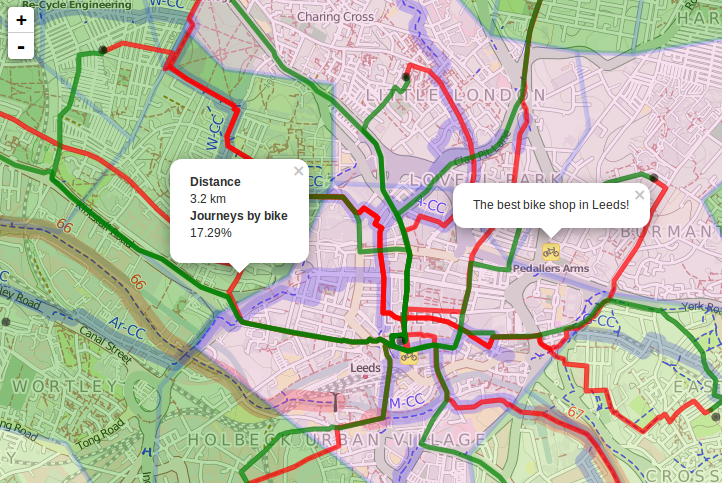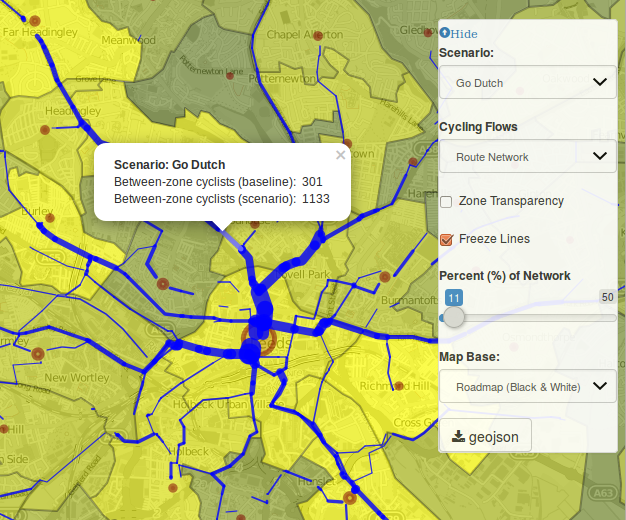Aggregating lines, Part I

Introduction

It’s been a busy 12 months but with the Geocomputation with R book nearing completion1 I’ve finally found some time to update my blog and do a bit of thinking, about the tangled topic of line aggregation.

Why aggregate lines?

The transport ‘flow’ on any particular segment of the transport networks is the aggregate (sum) of trips that pass through it1. Finding the flow across a transport network based on input data composed of individual routes, is therefore an aggregation problem. It requires a more complex solution than that provided by the aggregate() function in the base R2 package stats, however, because the geometry of the output LINESTRINGs will be fundamentally different than the input LINESTRINGS of the routes: a route network is composed of many small way segments, but a route is a single long LINESTRING.

Aggregating lines with overline() MKI

Creating such a route network, with aggregated values per segment, is the problem that the overline() function in the R package stplanr was designed to solve (see a 3.5 yr-old question on gis.stackexchange.com for more context).

The function works well and is the basis of the Route Network layer (MSOA) in the Propensity to Cycle Tool (PCT). In fact it was developed precisely for this purpose, as illustrated in the image below, which shows a common visualization/analysis problem encountered by transport researchers when working with multiple routes: overlapping routes are not easy to identify from non-overlapping routes: notice the red lines in the centre of Leeds in the image look the same as the red lines on the outskirts, despite representing much more movement.Figure 1: Demonstration of the need for line aggregation from a very early version of the Propensity to Cycle Tool, developed at the ‘Hack my Route’ data dive at ODI Leeds.

To overcome the problem overline() was born (credit to Barry Rowlingson who wrote the foundations of the function), and it works (on a tiny dataset of 2 lines) as follows:

library(stplanr)
sl_sp = routes_fast[2:3, ]
sl_sp@data = data.frame(value = c(2, 5))
plot(sl_sp, lwd = 9, col = "yellow")
rnet_sp = overline(sl_sp, attr = "value")
plot(rnet_sp, add = TRUE, lwd = rnet_sp$value)Figure 2: Illustration of the overline() function for line aggregation in action on a tiny example dataset. Note the left-most line is wider than the two lines that feed into it, demonstrating how line aggregation can be used to estimate flow on a road network. The utility of such a function is illustrated in the figure below, which shows the original route network layer of the PCT in action over a similar area of Leeds:Figure 3: Early version of the route network layer in the Propensity to Cycle Tool. This works great and the resulting network is used for strategic network planning: you can download route network data in the ‘Region data’ tab of the PCT3. The route network data for Leeds can, for example, be downloaded as a .geojson file from here. But there are some issues: the function works on the older SpatialLinesDataFrame class defined in the sp package4. This data class has been superseded by the simpler sf class in the sf package, which is faster than sp for some (if not many) operations. Another issue with overline() is that in some cases when 2 lines meet, the resulting aggregated line can be longer than it should be. So there are performance and functionality issues to address. Rather than solve them all here, this post sets-out the issue using reproducible code and suggests next steps for a new overline() function (or perhaps just an updated overline.sf() function which currently just wraps overline.sp()). Aggregating lines with sf Rather that starting from scratch and writing a geographic algorithm from the ground-up, we will start by exploring solutions provided by existing packages, notably sf, which provides an interface to the GEOS library. Let’s start simple, with just 2 lines, which have an associated amount of flow (with illustrative values of 2 and 5 in this case): library(stplanr) sl = routes_fast_sf[2:3, ] sl$value = c(2, 5)

These lines clearly have a decent amount of overlap, which can be extracted using the function st_intersection():

sl_intersection = sf::st_intersection(sl[1, ], sl[2, ])
plot(sl$geometry, lwd = 9, col = sf::sf.colors(2, alpha = 0.5)) plot(sl_intersection, add = TRUE)Figure 4: Illustration of the results of st_intersection Furthermore, we can find the aggregated value associated with this new segment as follows: sl_intersection$value = sum(sl_intersection$value, sl_intersection$value.1)

We still do not have a full route network composed of 3 non-overlapping lines, however: the original lines need to be ‘clipped’ so that they do not overlap with sl_intersection. This can be done as follows:

sl_seg1 = sf::st_difference(sl[1, ], sl_intersection)
sl_seg2 = sf::st_difference(sl[2, ], sl_intersection)
plot(sl$geometry, lwd = 9, col = sf::sf.colors(2, alpha = 0.5)) plot(sl_seg1, add = TRUE) plot(sl_seg2, add = TRUE)We now have all the geographic components needed for a route network. The only remaining task is to combine them, using rbind, right? Not quite: the following command fails: rnet = rbind(sl_seg1, sl_seg2, sl_intersection) ## Error in rbind.data.frame(...): numbers of columns of arguments do not match Lesson: we need to be more careful in isolating the value to aggregate. We will therefore run the previous stages again, but with attrib set to the attribute we would like to aggregate over (value in this case): attrib = "value" attrib1 = paste0(attrib, ".1") sl_intersection = sf::st_intersection(sl[1, attrib], sl[2, attrib]) sl_intersection[[attrib]] = sl_intersection[[attrib]] + sl_intersection[[attrib1]] sl_intersection[[attrib1]] = NULL That leaves us with a ‘clean’ object that only has a value (7) for the attribute column name we want (value). On this basis we can proceed to create the other segments, keeping only the column we’re interested in. To save time and typing, we’ll create both segments in a single command: sl_seg = sf::st_difference(sl[attrib], sf::st_geometry(sl_intersection)) rnet = rbind(sl_intersection, sl_seg) It worked! Now we’re in a position to plot the resulting route network, with ‘width’ proportional to the flow along each segment: plot(rnet, lwd = rnet$value)Figure 5: Illustration of a route network generated with sf functions.

A benchmark

To test that the method is fast, or is at least not slower than the original overline() function, at least for this task, we’ll package-up the method in a new function:

overline_sf2 = function(sl, attrib) {
attrib = "value"
attrib1 = paste0(attrib, ".1")
sl_intersection = sf::st_intersection(sl[1, attrib], sl[2, attrib])
sl_intersection[[attrib]] = sl_intersection[[attrib]] + sl_intersection[[attrib1]]
sl_intersection[[attrib1]] = NULL
sl_seg = sf::st_difference(sl[attrib], sf::st_geometry(sl_intersection))
rnet = rbind(sl_intersection, sl_seg)
return(rnet)
}

If you are new to scripts/algorithms/functions, it may be worth taking a look at the new Algorithms chapter in our near-complete book that teaches a range of geographic methods that use R5. Now the method has been put in a function, we can compare its performance with the per-existing overline() function for comparison:

bench::mark(check = F,
overline.sp = overline(sl_sp, attrib = "value"),
overline.sf = overline(sl, attrib = "value"),
overline_sf2 = overline_sf2(sl, attrib = "value")
)
## # A tibble: 3 x 10
##   expression      min   mean median    max itr/sec mem_alloc  n_gc n_itr
##   <bch:expr>   <bch:> <bch:> <bch:> <bch:>     <dbl> <bch:byt> <dbl> <int>
## 1 overline.sp  14.7ms 15.9ms 15.1ms 27.7ms      62.8    71.3KB     3    26
## 2 overline.sf  18.3ms 19.3ms 18.6ms 32.8ms      51.9   369.9KB     3    23
## 3 overline_sf2 12.2ms 12.7ms 12.6ms 18.3ms      78.5     125KB     6    32
## # … with 1 more variable: total_time <bch:tm>

The results are not Earth-shattering: the new function seems to be around the same speed as the original, if a little faster. This is great news, but remember: the new function only works on 2 lines so is much simpler. More work needed!

Next steps

The next step is to generalist this method so it works for many (potentially thousands) of lines in a way that scales, something that should help on the visualization side, a topic that attracts much interest (see for example this gis.stackexchange post on the subject and, more broadly, a recent article showing how to make animated maps by Jakub Nowosad). There is also work to do on performance but, as Donald Knuth said6:

premature optimization is the root of all evil (or at least most of it) in programming

So a more complete overline.sf() function is needed. That will (hopefully) be the topic of the next blog post.

1. Any input on that still welcome - see the contributing section for more.